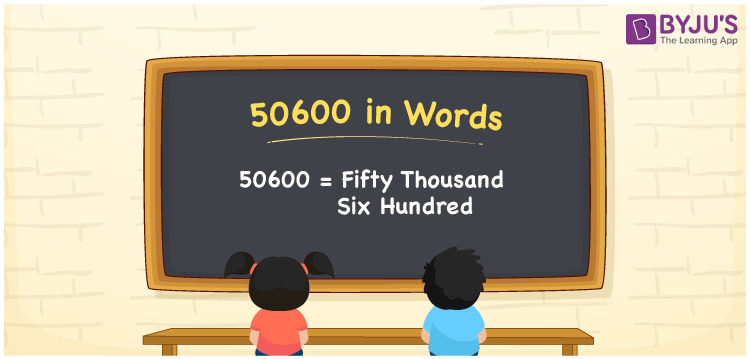# 50600 in Words

50600 in words is written as Fifty thousand six hundred. In both the International System of Numerals and the Indian System of Numerals, 50600 in words is written as Fifty thousand six hundred. The number 50600 is a Cardinal Number as it represents some quantity. For example, “that laptop costs 50600 rupees”.

 50600 in Words Fifty thousand six hundred Fifty thousand six hundred in Number 50600

## 50600 in English Words

50600 in English words is read as “Fifty thousand six hundred.”## How to Write 50600 in Words?

To write 50600 in words, we shall use the place value chart. In the place value chart, put 5 in the ten thousands, 0 in the thousands, 6 in the hundreds and 0 in the tens and the ones, respectively. Let us make a place value chart to write the number 50600 in words.

 Ten Thousands Thousands Hundreds Tens Ones 5 0 6 0 0

Thus, we can write the expanded form as

5 × Ten Thousand + 0 × Thousand + 6 × Hundred + 0 × Ten + 0 × One

= 5 × 10000 + 0 × 1000 + 6 × 100 + 0 × 10 + 0 × 1

= 50000 + 0 + 600 + 0 + 0

= 50600

= Fifty thousand six hundred.

50600 is a natural number, the successor of 50599 and the predecessor of 50601.

50600 in words – Fifty thousand six hundred

• Is 50600 an odd number? – No
• Is 50600 an even number? – Yes
• Is 50600 a perfect square number? – No
• Is 50600 a perfect cube number? – No
• Is 50600 a prime number? – No
• Is 50600 a composite number? – Yes

## Frequently Asked Questions on 50600 in Words

Q1

### How to write 50600 in words?

50600 in words is written as Fifty thousand six hundred.
Q2

### How to write 50600 in the International and Indian System of Numerals?

In both, the system of numerals, 50,600 in words, is written as Fifty thousand six hundred.
Q3

### How to write 50600 in a place value chart?

In the place value chart, write 5 in the ten thousands place, 0 in the thousands, 6 in the hundreds place and again 0 in the tens and the ones place respectively.